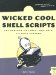6 Validating Floating-Point Input

#6 Validating Floating-Point Input

Upon first glance, the process of validating a floating-point (or "real") value within the confines and capabilities of a shell script might seem daunting, but consider that a floating-point number is only two integers separated by a decimal point. Couple that insight with the ability to reference a different script inline ( validint ), and you can see that the floating-point validation test is surprisingly short.

The Code

#!/bin/sh # validfloat -- Tests whether a number is a valid floating-point value. # Note that this script cannot accept scientific (1.304e5) notation. # To test whether an entered value is a valid floating-point number, we # need to split the value at the decimal point. We then test the first part # to see if it's a valid integer, then test the second part to see if it's a # valid >=0 integer, so -30.5 is valid, but -30.-8 isn't. . validint   # Bourne shell notation to source the validint function validfloat() {   fvalue=""   if [ ! -z \$(echo \$fvalue  sed 's/[^.]//g') ] ; then     decimalPart="\$(echo \$fvalue  cut -d. -f1)"     fractionalPart="\$(echo \$fvalue  cut -d. -f2)"     if [ ! -z \$decimalPart ] ; then       if ! validint "\$decimalPart" "" "" ; then         return 1       fi     fi     if [ "\${fractionalPart%\${fractionalPart#?}}" = "-" ] ; then       echo "Invalid floating-point number: '-' not allowed \         after decimal point" >&2       return 1     fi     if [ "\$fractionalPart" != "" ] ; then       if ! validint "\$fractionalPart" "0" "" ; then         return 1       fi     fi     if [ "\$decimalPart" = "-" -o -z "\$decimalPart" ] ; then       if [ -z \$fractionalPart ] ; then         echo "Invalid floating-point format." >&2 ; return 1       fi     fi   else     if [ "\$fvalue" = "-" ] ; then       echo "Invalid floating-point format." >&2 ; return 1     fi     if ! validint "\$fvalue" "" "" ; then       return 1     fi   fi   return 0 }

Running the Script

If no error message is produced when the function is called, the return code is 0, and the number specified is a valid floating-point value. You can test this script by appending the following few lines to the end of the code just given:

if validfloat  ; then   echo " is a valid floating-point value" fi exit 0

The Results

\$  validfloat 1234.56  1234.56 is a valid floating-point value \$  validfloat -1234.56  -1234.56 is a valid floating-point value \$  validfloat -.75  -.75 is a valid floating-point value \$  validfloat -11.-12  Invalid floating-point number: '-' not allowed after decimal point \$  validfloat 1.0344e22  Invalid number format! Only digits, no commas, spaces, etc.
 Debugging the debugging If you see additional output at this point, it might be because you added a few lines to test out validint earlier, but forgot to remove them when you moved on to this script. Simply go back to validint and ensure that the last few lines that run the function are commented out or deleted.

Hacking the Script

A cool additional hack would be to extend this function to allow scientific notation, as demonstrated in the last example. It wouldn't be too difficult. You'd test for the presence of 'e' or 'E' and then split the result into three segments: the decimal portion (always a single digit), the fractional portion, and the power of ten. Then you just need to ensure that each is a validint .Wicked Cool Shell Scripts
ISBN: 1593270127
EAN: 2147483647
Year: 2004
Pages: 150
Authors: Dave Taylor

Similar book on Amazon